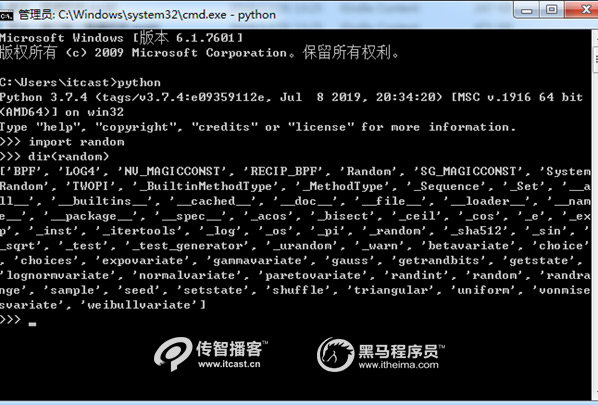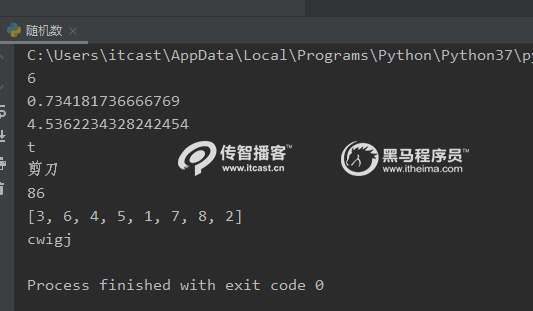﻿ Python中random函数的用法[python学习分享]

# Python中random函数的用法[python学习分享]

random模块是python自带的模块，用于生成随机数，需要注意的是random()是不能直接访问的，需要导入 random 模块，然后通过 random 静态对象调用该方法。

>>> import random
>>> dir(random)# 导入random工具包
import random
# 产生1到10的一个整数型随机数
print(random.randint(1, 10))
# 产生0到1之间的随机浮点数
print(random.random())
# 产生1.1到5.4之间的随机浮点数,区间可以不是整数
print(random.uniform(1.1, 5.4))
# 从序列中随机选取一个元素
print(random.choice('tomorrow'))
print(random.choice(['剪刀', '石头', '布']))
# 生成从1到100的间隔为2的随机整数
print(random.randrange(1, 100, 1))
# 将序列a中的元素顺序打乱
a=[1, 2, 3, 4, 5, 6, 7, 8]
random.shuffle(a) print(a)
# 多个字符中选取指定数量的字符组成新字符串
print(''.join(random.sample(['z', 'y', 'x', 'w', 'v', 'u', 't', 's','r', 'q', 'p', 'o','n', 'm',
'l', 'k',  'j', 'i', 'h', 'g', 'f', 'e', 'd', 'c', 'b', 'a'], 5)))